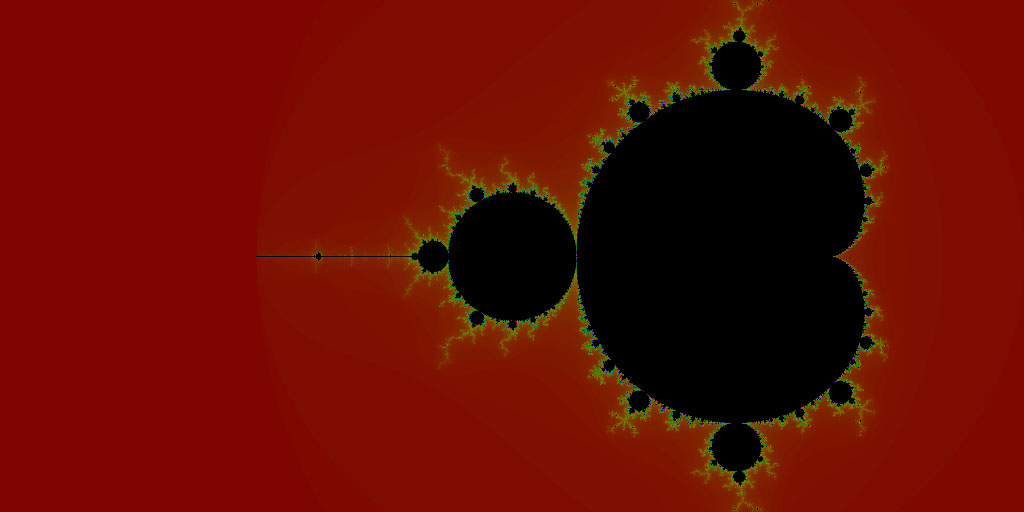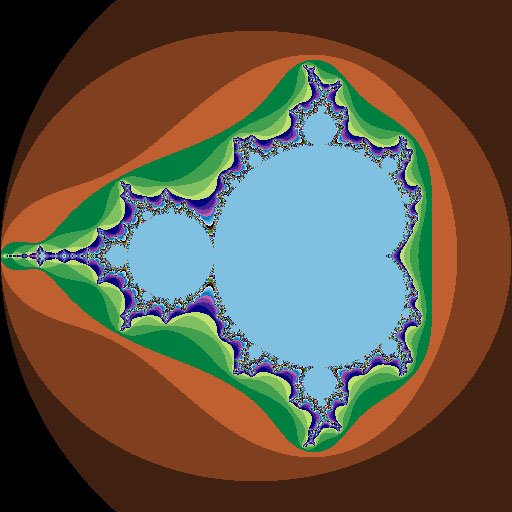Open in App
Not now

# Mandelbrot Fractal Set visualization in Python

• Last Updated : 03 Oct, 2018

Fractal:
A fractal is a curve or geometrical figure, each part of which has the same statistical character as the whole. They are useful in modeling structures (such as snowflakes) in which similar patterns recur at progressively smaller scales, and in describing partly random or chaotic phenomena such as crystal growth and galaxy formation.

In simpler words, a fractal is a never-ending pattern. Fractals are infinitely complex patterns that are self-similar across different scales. They are created by repeating a simple process over and over in an ongoing feedback loop. Driven by recursion, fractals are images of dynamic systems – the pictures of Chaos.
Geometrically, they exist in between our familiar dimensions. Fractal patterns are extremely familiar since nature is full of fractals. For instance: trees, rivers, coastlines, mountains, clouds, seashells, hurricanes, etc. Abstract fractals – such as the Mandelbrot Set – can be generated by a computer calculating a simple equation over and over.

Mandelbrot set:
The Mandelbrot set is the set of complex numbers c for which the functiondoes not diverge when iterated from z=0, i.e., for which the sequence, etc., remains bounded in absolute value. In simple words, Mandelbrot set is a particular set of complex numbers which has a highly convoluted fractal boundary when plotted.

Installation of needed Python modules:

```pip install pillow
pip install numpy```

Code #1:

 `# Python code for Mandelbrot Fractal ` ` `  `# Import necessary libraries ` `from` `PIL ``import` `Image ` `from` `numpy ``import` `complex``, array ` `import` `colorsys ` ` `  `# setting the width of the output image as 1024 ` `WIDTH ``=` `1024` ` `  `# a function to return a tuple of colors ` `# as integer value of rgb ` `def` `rgb_conv(i): ` `    ``color ``=` `255` `*` `array(colorsys.hsv_to_rgb(i ``/` `255.0``, ``1.0``, ``0.5``)) ` `    ``return` `tuple``(color.astype(``int``)) ` ` `  `# function defining a mandelbrot ` `def` `mandelbrot(x, y): ` `    ``c0 ``=` `complex``(x, y) ` `    ``c ``=` `0` `    ``for` `i ``in` `range``(``1``, ``1000``): ` `        ``if` `abs``(c) > ``2``: ` `            ``return` `rgb_conv(i) ` `        ``c ``=` `c ``*` `c ``+` `c0 ` `    ``return` `(``0``, ``0``, ``0``) ` ` `  `# creating the new image in RGB mode ` `img ``=` `Image.new(``'RGB'``, (WIDTH, ``int``(WIDTH ``/` `2``))) ` `pixels ``=` `img.load() ` ` `  `for` `x ``in` `range``(img.size[``0``]): ` ` `  `    ``# displaying the progress as percentage ` `    ``print``(``"%.2f %%"` `%` `(x ``/` `WIDTH ``*` `100.0``))  ` `    ``for` `y ``in` `range``(img.size[``1``]): ` `        ``pixels[x, y] ``=` `mandelbrot((x ``-` `(``0.75` `*` `WIDTH)) ``/` `(WIDTH ``/` `4``), ` `                                      ``(y ``-` `(WIDTH ``/` `4``)) ``/` `(WIDTH ``/` `4``)) ` ` `  `# to display the created fractal after  ` `# completing the given number of iterations ` `img.show() `

Output:Code #2:

 `# Mandelbrot fractal ` `# FB - 201003254 ` `from` `PIL ``import` `Image ` ` `  `# drawing area ` `xa ``=` `-``2.0` `xb ``=` `1.0` `ya ``=` `-``1.5` `yb ``=` `1.5` ` `  `# max iterations allowed ` `maxIt ``=` `255`  ` `  `# image size ` `imgx ``=` `512` `imgy ``=` `512` `image ``=` `Image.new(``"RGB"``, (imgx, imgy)) ` ` `  `for` `y ``in` `range``(imgy): ` `    ``zy ``=` `y ``*` `(yb ``-` `ya) ``/` `(imgy ``-` `1``)  ``+` `ya ` `    ``for` `x ``in` `range``(imgx): ` `        ``zx ``=` `x ``*` `(xb ``-` `xa) ``/` `(imgx ``-` `1``)  ``+` `xa ` `        ``z ``=` `zx ``+` `zy ``*` `1j` `        ``c ``=` `z ` `        ``for` `i ``in` `range``(maxIt): ` `            ``if` `abs``(z) > ``2.0``: ``break` `            ``z ``=` `z ``*` `z ``+` `c ` `        ``image.putpixel((x, y), (i ``%` `4` `*` `64``, i ``%` `8` `*` `32``, i ``%` `16` `*` `16``)) ` ` `  `image.show() `

Output:My Personal Notes arrow_drop_up
Related Articles